Informatiker Board » Themengebiete » Theoretische Informatik » N = Np » Hallo Gast [Anmelden|Registrieren]
Autor
 Beitrag
 Anonycat90 Grünschnabel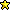Dabei seit: 28.07.2022 Beiträge: 1Meine Frage: N = PN I discovered a wonderful algorithm and wanted to share it with you all. First we need to assume that string theory fully explains the phenomenon of W boson superheavy. We use the mass operator of string theory to correct the standard mass of the W boson. If the magnitude of the correction can eliminate 7 confidence intervals, it proves that the string theory is based on experimental facts. At this time, we need to review the algorithm of the Hamiltonian graph I wrote earlier, which was mentioned in my previous blog post on Facebook. We need to construct the topology of a simple "1+2+3" instance, which will be in the shape of a Möbius strip. First, we need to know that this structure is the simplest instance of a Hamiltonian graph. After that, we construct the conjecture of the 24 Umbral moonshine conjecture. It should be noted that the 24 Umbral moonshine conjecture here are all in line with Ramanujan's third-order simulation equation. If we use the Möbius strip of the "1+2+3" topology, we can easily slide a rule that a third-order equation must correspond to a first-order equation and a second-order equation. In this case, we can simplify it like this The third-order Ramanujan simulation equation, at this time, we can complete the simulation of 24 string effects through the mass operator of string theory. I think boson quantum computers are not the future, but the present. Meine Ideen: N = NP Anonycat90 hat dieses Bild (verkleinerte Version) angehängt: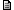28.07.2022 20:52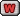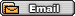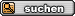Gehe zu: Bitte wählen: -------------------- Themengebiete-- Theoretische Informatik---- formale Sprachen---- Automatentheorie---- Berechenbarkeits- und Komplexitätstheorie---- Logik-- Praktische Informatik---- Algorithmen---- Softwaretechnik---- Datenbanken-- Technische Informatik-- übergreifende Themen---- Künstliche Intelligenz---- Informatik und Gesellschaft-- Informatik in der Schule-- Sonstige FragenSonstiges-- Off-Topic-- Ankündigungen
 Informatiker Board » Themengebiete » Theoretische Informatik » N = Np